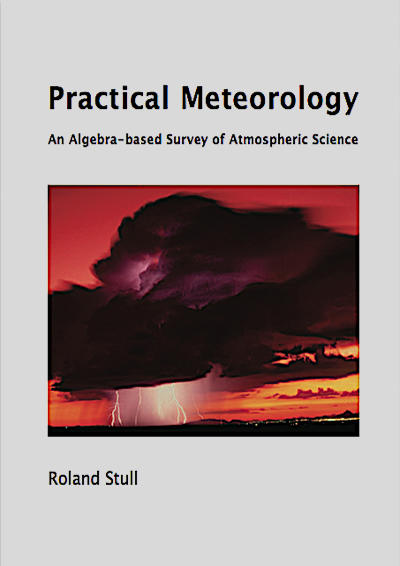Springe direkt zu Inhalt

# Practical Meteorology

## An Algebra-based Survey of Atmospheric SciencePractical Meteorology

Link zum Volltext

This book is designed for students and professionals who want to understand and apply basic meteorological concepts, but who don't need to derive equations.

To make this book accessible to more people, equations have been converted into algebra. With algebraic approximations to the atmosphere, you can see the physical meaning of each term and you can plug in numbers to get usable answers.

No previous knowledge of meteorology is needed -- the author starts from the basics. Your background should include algebra, trig, and classical physics. This book could serve the fields of Atmospheric Science, Meteorology, Environmental Science, Engineering, Air Quality, Climatology, and Geography.

This book is designed to be both a textbook and a reference. As a textbook, the end of each chapter includes extensive homework exercises in categories inspired by Bloom's taxonomy of learning actions: "Broaden Knowledge & Comprehension"; "Apply"; "Evaluate & Analyze"; and "Synthesize".

Although a hand calculator can be used for some of the homework exercises, other exercises are best solved on a computer spreadsheet such as Excel or using a mathematical program such as MATLAB, R, Mathematica, or Maple. I used Excel for my Sample Applications and most of my graphs.